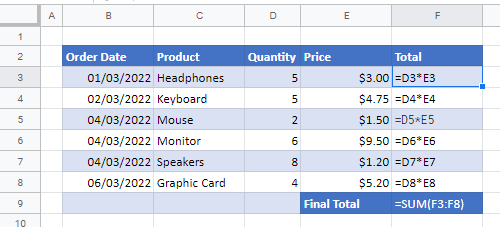# What Do the Symbols (&,\$,{, etc.) Mean in Formulas? – Excel & Google Sheets

Last updated on September 21, 2023

This tutorial explains what different symbols mean in formulas in Excel and Google Sheets.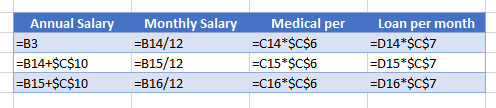Excel is essentially used for keeping track of data and using calculations to manipulate this data. All calculations in Excel are done by means of formulas, and all formulas are made up of different symbols or operators, depending on what function the formula is performing.

## Equal Sign (=)

The most commonly used symbol in Excel is the equal (=) sign. Every single formula or function used has to start with equals to let Excel know that a formula is being used. If you wish to reference a cell in a formula, it has to have an equal sign before the cell address. Otherwise, Excel just shows the cell address as standard text.

In this example, if you type (1) =B2 in cell D2, it returns a value of (2) 10. However, typing only (3) B3 into cell D3 just shows B3 in the cell, and there is no reference to the value 20.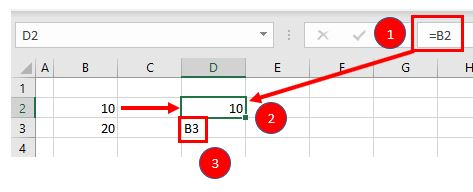## Standard Operators

The next most common symbols in Excel are the standard operators as used on a calculator: plus (+), minus (), multiplication (*) and division (/). Note that the multiplication sign is not the standard multiplication sign (×) but is depicted by an asterisk (*) while the division sign is not the standard division sign (÷) but is depicted by the forward slash (/).

An example of a formula using addition and multiplication is shown below:

`=B1+B2*B3`

## Order of Operations and Adding Parentheses

In the formula shown above, B2*B3 is calculated first, as in standard mathematics. The order of operations is always multiplication before addition. However, you can adjust the order of operations by adding parentheses (round brackets) to the formula; any calculations between these parentheses would then be done first before the multiplication. Parentheses, therefore, are another example of symbols used in Excel.

`=(B1+B2)*B3`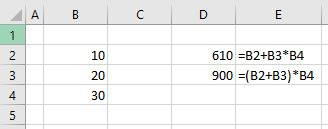In the example shown above, the first formula returns a value of 610 while the second formula (using parentheses) returns 900.

Parentheses are also used with all Excel functions. For example, to sum B3, B4, and B5 together, you can use the SUM Function where the range B3:B5 is contained within parentheses.

`=SUM(B3:B5)`

## Colon (:) to Specify a Range of Cells

In the formula used above, the parentheses contain the cell range which the SUM Function needs to add together. This cell range is expressed with a colon (:) where the first cell reference (B3) is the cell address of the first cell included in the range of cells to add together, while the second cell reference (B5) is the cell address of the last cell included in the range.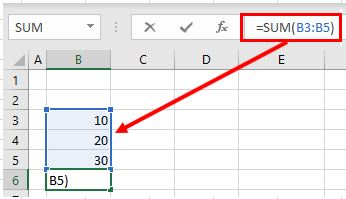## Dollar Symbol (\$) in an Absolute Reference

A particular useful and common symbol used in Excel is the dollar sign within a formula. Note that this does not indicate currency; rather, it’s used to “fix” a cell address in place in order that a single cell can be used repetitively in multiple formulas by copying formulas between cells.

`=C6*\$C\$3`

By adding a dollar sign (\$) in front of the column header (C) and the row header (3), when copying the formula down to Rows 7–15 in the example below, the first part of the formula (e.g., C6) changes according to the row it is copied down to while the second part of the formula (\$C\$3) stays static always enabling the formula to refer to the value stored in cell C3.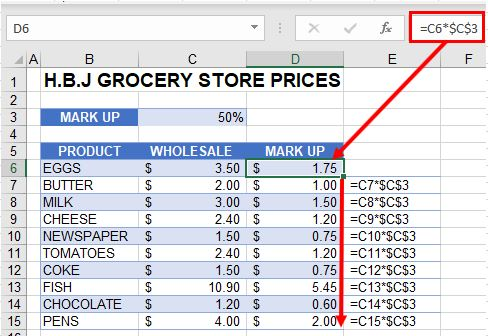See: Cell References and Absolute Cell Reference Shortcut for more information on absolute references.

## Exclamation Point (!) to Indicate a Sheet Name

The exclamation point (!) is critical if you want to create a formula in a sheet and include a reference to a different sheet.

`=SUM(Sheet1!B2:B4)`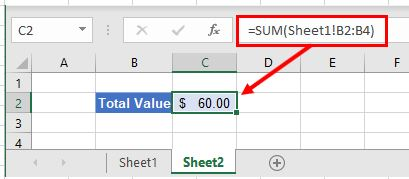### Easy Excel Automation## Square Brackets [ ] to Refer to External Workbooks

Excel uses square brackets to show references to linked workbooks. The name of the external workbook is enclosed in square brackets, while the sheet name in that workbook appears after the brackets with an exclamation point at the end.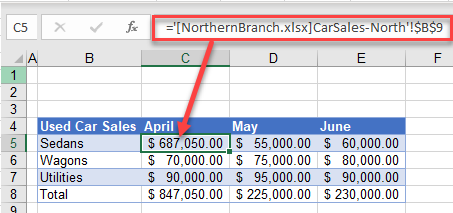## Comma (,)

The comma has two uses in Excel.

### Refer to Multiple Ranges

If you wish to use multiple ranges in a function (e.g., the SUM Function), you can use a comma to separate the ranges.

`=SUM(B2:B3, B6:B10)`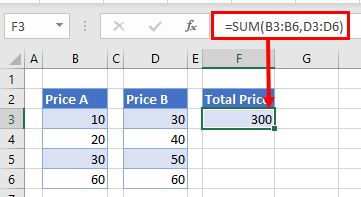### Separate Arguments in a Function

Alternatively, some built in Excel functions, like VLOOKUP, have multiple arguments which are usually separated with commas. (These can also be semicolons, depending on the function syntax.)

`=VLOOKUP(H5, B6:E15, 4, FALSE)`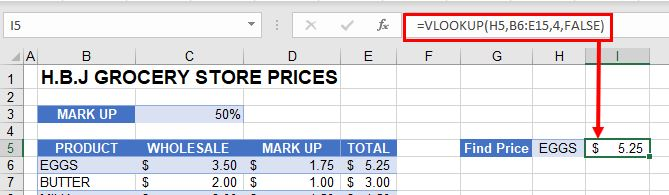## Curly Brackets { } in Array Formulas

Curly brackets are used in array formulas. An array formula is created by pressing the CTRL + SHIFT + ENTER keys together when entering a formula.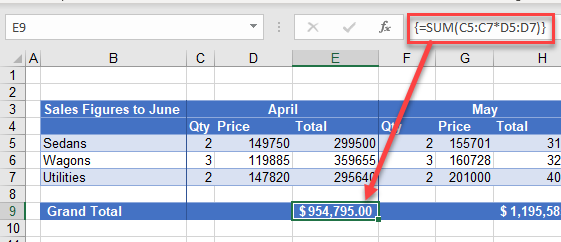## Other Important Symbols

Symbol Description Example
% Percentage =B2%
^ Exponential operator =B2^B3
& Concatenation =B2&B3
> Greater than =B2>B3
< Less than =B2<B3
>= Greater than or equal to =B2>=B3
<= Less than or equal to =B2<=B3
<> Not equal to =B2<>B3

## Symbols in Formulas in Google Sheets

The symbols used in Google Sheets are identical to those used in Excel.